# Semi-algebraic set

semi-analytic set.

A semi-algebraic set in(or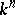, whereis a real closed field) is a set that can be given by finitely many polynomial equalities and inequalities. More precisely, for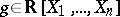, let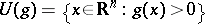. Thenis semi-algebraic if it belongs to the smallest Boolean ring of subsets ofcontaining all the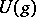.

A semi-analytic set is, by definition, a set in a real-analytic manifold which can be locally described by finitely many analytic equalities and inequalities.

The Tarski–Seidenberg theorem asserts the existence of a decision procedure (cf. also Decidable set) for deciding the truth of any elementary sentence built up from finitely many polynomial inequalities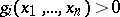, the connectives "and" , "or" and "not" , and the quantifiers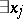,. Two precise formulations are: 1) Letbe a semi-algebraic set andthe projection onto the last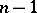coordinates. Then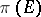is semi-algebraic. 2) Letbe a finite sentence built up from inequalitiesand the connectives "and" , "or" and "not" (such a sentence is called a polynomial relation). Let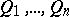be a series of quantifiers of the formor. There is an algorithm for finding a polynomial relationsuch that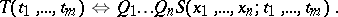It follows from the Tarski–Seidenberg theorem that the image under a polynomial mappingof a semi-algebraic set is semi-algebraic. Indeed, this is equivalent.

The image of a semi-analytic set under an analytic mapping is not necessarily semi-analytic. A subanalytic set on a real-analytic manifold is, by definition, a set that is locally the image of a semi-analytic set under an analytic mapping. The points of a subanalytic set at which it is not semi-analytic form a subanalytic set, cf. [a2].

The closure of a semi-algebraic (respectively, semi-analytic or subanalytic) set is again semi-algebraic (respectively, semi-analytic or subanalytic).

The image of a semi-algebraic (respectively, subanalytic) set under an algebraic (respectively, analytic) mapping is a semi-algebraic (respectively, subanalytic) set.

Finally, a semi-algebraic (respectively, semi-analytic or subanalytic) subset of a smooth algebraic (respectively, analytic or analytic) variety admits a smooth stratification, whose strata are semi-algebraic (respectively, semi-analytic or subanalytic) (and smooth).

How to Cite This Entry:
Semi-algebraic set. Encyclopedia of Mathematics. URL: http://encyclopediaofmath.org/index.php?title=Semi-algebraic_set&oldid=23974